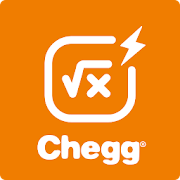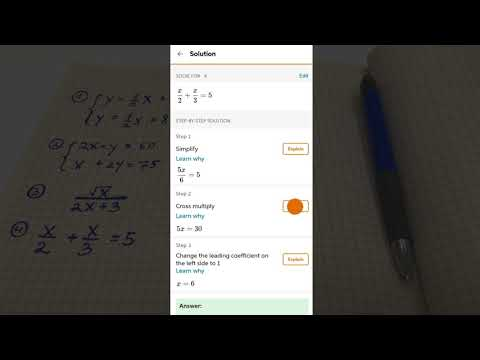Chegg Math Solver - guided math problem solverEveryone
169
Offers in-app purchasesChegg Math Solver is more than a calculator.👍 👍 👍It digs into each step of your algebra, pre-calculus, or calculus math problems to learn how and why they were solved that way.

Photo math equation transform. It’s easy to input a math problem in Chegg Math Solver with the photo math transform feature. You simply need to scan a math equation and take a photo with the camera of your mobile device. Chegg Math Solver will transform your photo into math equation and show you the step-by-step solution in a few seconds. The photo math magic save your efforts to input complicated math problems from the calculator.

Chegg Math Solver is for every student. No matter you are struggling with your math homework, preparing for your math final exams, or just want to understand math problems in depth, start Chegg Math Solver today and try the photo math transform function. Chegg Math Solver is the trusted math help to make math easier.

Chegg Math Solver is for every parent. It explains the solution of complicated math problems step by step, and makes them easier to understand. Start Chegg Math Solver today to help your children get better grades.

Leverage the photo math magic of Chegg Math Solver to help with your schoolwork in:
- Pre-algebra: fractions, linear equations, negative numbers, linear inequalities, powers and roots
- Pre-calculus: exponential and logarithmic functions, adding matrices, multiplying matrices, matrix inverses and determinants
- Calculus: fundamental derivatives, general derivatives, curve sketching, fundamental integrals, general integrals
- Linear algebra: matrix operations, inverse matrices, determinants, characteristic polynomial, eigenvalues

Chegg Math Solver requires a subscription.
- Your subscription will automatically renew and your Google Play account will be charged for renewal 24-hours prior to the end of your current subscription period.

Download Chegg Math Solver today! Use the photo math transform feature to solve your math problems on the go!
Collapse

Review Policy
3.6
169 total
5
4
3
2
1

What's New

Collapse

Updated
June 6, 2019
Size
41M
Installs
10,000+
Current Version
1.6.1
Requires Android
5.0 and up
Content Rating
Everyone
Interactive Elements
Digital Purchases
In-app Products
\$0.99 - \$9.99 per item
Permissions
Offered By
Chegg, Inc.
Developer
Chegg Inc. 3990 Freedom Circle, Santa Clara, CA 95054-1204